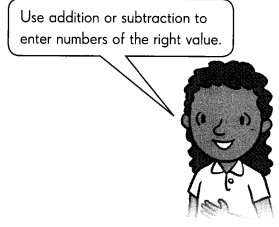# Math in Focus Grade 4 Chapter 1 Answer Key Place Value of Whole Numbers

Go through the Math in Focus Grade 4 Workbook Answer Key Chapter 1 Place Value of Whole Numbers to finish your assignments.

## Math in Focus Grade 4 Chapter 1 Answer Key Place Value of Whole Numbers

Math Journal

Question 1.
Kim wrote these statements about the three numbers shown here.
Do you agree? Explain why or why not.
3,869 is less than 85,945.
85,691 is greater than 85,945.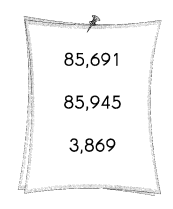The above-given statements are:
1. 3,869 is less than 85,945.
2. 85,691 is greater than 85,945.
The first statement is correct.
The second statement is wrong.
The first statement is correct because we are comparing numbers with their place values.
In Mathematics, place value charts help us to make sure that the digits are in the correct places. To identify the positional values of numbers accurately, first, write the digits in the place value chart and then write the numbers in the usual and the standard form.
Rules for comparing numbers:
There are certain rules, based on which it becomes easier to compare numbers. These rules are:
* Numbers with more digits
* Numbers starting with a larger digit
Rule 1: Numbers with more digits
– When we compare numbers, then check if both the numbers are having the same number of digits or not. If a number has more digits, then it is greater than the other number.
Examples:
– 33 > 3
– 400 > 39
– 5555 > 555
– 10000 > 9999
Rule 2: Numbers starting with a larger digit
This rule is applicable when two numbers are having the same number of digits. In such cases, we need to check the digit at the leftmost place, whichever is greater. Therefore, the number with a greater digit at the leftmost place of the number is greater than the other number.
Examples:
* 323>232 [323 is greater than 232]
* 343<434 [343 is less than 434]
Thus, in the above examples, we can see that, when we compare the two numbers, though the number of digits is the same, one number is greater than/less than the other number.
Now according to the above rules, we check statement 1:
1. 3,869 is less than 85,945.
– In this statement, two numbers are given. The first number is having fewer digits than the second number so here rule 1 is applicable.
absolutely, 3,869 < 85,945
This can be written in another way:
85,945 > 3,869.
Now we check for statement 2:
2. 85,691 is greater than 85,945.
Here the same number of digits are having so rule 2 is applicable.
– 85,691 and 85,945 both the numbers have an equal number of digits, therefore, we will compare the left-most digit of both the numbers.
– As we can see the first two digits are the same for both the numbers, thus we need to compare the next left-most digit of both numbers.
6 < 9
Here it is a wrong statement because 6 is not greater than 9. So the second statement is wrong.

Question 2.
Sam continued this number pattern.
Do you agree? Explain why or why not.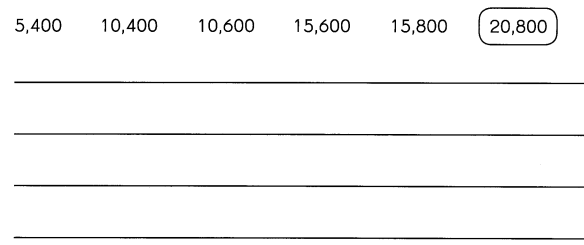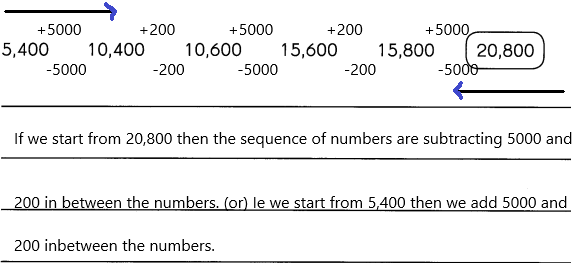For number patterns there are certain rules:
To create a complete pattern, there are a set of rules to be considered. To apply the rule, we need to understand the nature of the sequence and the difference between the two successive numbers. It takes some amount of guesswork and checking to see whether the rule works throughout the whole series.
There are two basic divisions to find out the rules in number patterns:
* When the numbers in the given pattern get larger, they are said to be in ascending order. These patterns usually involve addition or multiplication.
* When the numbers in the given pattern get smaller, they are said to be in descending order. These patterns usually involve subtraction or division.
So the number pattern is correct.

Question 3.

Example
45,870
1. The digit 5 is in the thousands place.
2. The value of the digit 7 is 70.
3. The digit in the hundreds place is 10 – 2.
4. The digit in the ten thousands place is 1 less than the digit in the thousands place.
5. The digit in the ones place is 0.

Number: _______
Clues: ______
– According to the clues, the number is 15,870
– Now in line number 4, the digit in the ten thousand places is 1 less than hundreds of places. So, 1 < 5. 5 is in the thousand places so I placed 5 in the thousands place.
– In line 3 the hundreds place is 8. In the above-example 10-2 is given which is 8. So I placed 8 in the hundreds place.
– In line 2 the number 70 is given, 70 is nothing but 7 tens so I placed 7 in the tens place.
– In line 5 directly given that one’s place is 0.

Challenging Practice

Complete.

A 5-digit number is made up of different digits that are all odd numbers.

Question 1.
What is the greatest possible number? ______
Explanation:
The largest 5 digit number that you can have when all digits are odd would be 99999.
that’s with no restrictions.
any other number you choose would have to be smaller.
the restrictions are:
the ten thousand digits are 3 times the one’s digit.
since the ten-thousands digit is fixed at 9, then the one’s digit has to be 3 because 3 * 3 = 9.
the thousands digit is 2 more than the hundreds digit.
since the thousands digit is fixed at 9, then the hundreds digit has to be 7 because 7 + 2 = 9.
that makes your number equal to 99793.
any other arrangement would force the ten-thousands digit to be something less than 9 or the thousands digit to be less than 9 which would result in a smaller number.

Question 2.
What is the value of the digit in the hundreds place? _____
Explanation:
The largest 5 digit number that you can have when all digits are odd would be 99999.
The place values of 99999 are:
9 is in the hundred thousand place.
9 is in the ten thousand place
9 is in the thousand place
9 is in the hundreds place
9 is in the tens place
9 is in the one’s place.

Continue the pattern.

Question 3.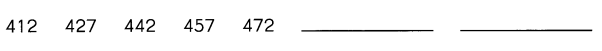Explanation:
A list of numbers that follow a certain sequence is known as patterns or number patterns. The different types of number patterns are algebraic or arithmetic patterns, geometric patterns, Fibonacci patterns and so on.
The above-given numbers are in the arithmetic pattern.
The arithmetic pattern is also known as the algebraic pattern. In an arithmetic pattern, the sequences are based on the addition or subtraction of the terms. If two or more terms in the sequence are given, we can use addition or subtraction to find the arithmetic pattern.
The numbers are 412  427  442  457  472  –  -. Now, we need to find the missing term in the sequence.
Here, we can use the addition process to figure out the missing terms in the patterns.
In the pattern, the rule used is “Add 15 to the previous term to get the next term”.
In the numbers given above, take the second term (427). If we add “15” to the second term (427), we get the third term 442.
Similarly, we can find the unknown terms in the sequence.
First missing term: The previous term is 472. Therefore, 472+15 = 487.
Second missing term: The previous term is 487. So, 487+15 = 502
Hence, the complete arithmetic pattern is 412  427   442   457   472   487   502.

Fill in the blanks.

Question 4.
What is 3 ten thousands + 14 tens + 16 ones? ______
Explanation:
In a number, the place (local) value of a non-zero digit is the value of this digit according to its position.
A number can have many digits, and each digit has a special place and value
ten thousand can be written as 10,000
tens can be written as 10
ones can be written as 1
3 x 10,000 = 30,000, 14 x 10 = 140 and 16 x 1 = 16
30 000 + 140 + 16 = 30 156.

Question 5.
7 thousands = _____ hundreds 10 tens
7 thousand=7 hundreds 10 tens
This can be written as:
7000=700*10
7 thousands=70 hundreds
7 thousand=700 tens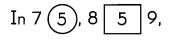Question 6.
what is the value of the digit 5 in the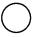? ____
Explanation:
In Mathematics, place value charts help us to make sure that the digits are in the correct places. To identify the positional values of numbers accurately, first, write the digits in the place value chart and then write the numbers in the usual and the standard form.
According to the above solution, 5 is in the thousands place.
5 thousands is nothing but 5000
So it can be written as 5000.

Question 7.
what is the value of the digit 5 in the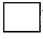? ____
Explanation:
In Mathematics, place value charts help us to make sure that the digits are in the correct places. To identify the positional values of numbers accurately, first, write the digits in the place value chart and then write the numbers in the usual and the standard form.
According to the above solution, 5 is in the tens place.
5 tens are nothing but 50
So it can be written as 50.

Question 8.
what is the difference between the answers in Exercises 6 and 7? ____
Explanation:
The place value is the position of each digit in a number. The place value of digits is determined as ones, tens, hundreds, thousands,ten-thousands and so on, based on their position in the number. For example, the place value of 1 in 1002 is thousands, i.e.1000.
The above-given number 75,859
7 is in the ten thousand place
5 is in the thousands of place
8 is in the hundreds place
5 is in the tens place
9 is in the one’s place.

Question 9.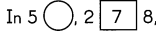, the difference between the values of the digits in the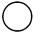and the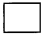is 8,930. What is the digit in the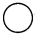?
The above-given number 5 X,278
We need to find out the X.
The difference betweenand=8,930
Already we know the value in the square that is 7
X-7=8,930
If we subtract 59,278 and 50,348 then we get 8,930
so 9 is in the ten thousand places.
Check which number we subtract from the given number to get 8,930.
If we kept 9 in the X place and subtract with the assumed number that is 50,348 then we get the given number 8,930. Likewise we need to think and process the problem.

Problem Solving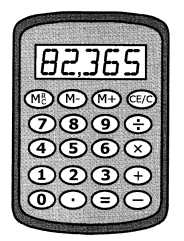The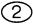button and the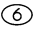button do not work.
Justin wants to enter the number 82,365.
Explain what he can do to key in the number. Give two solutions.